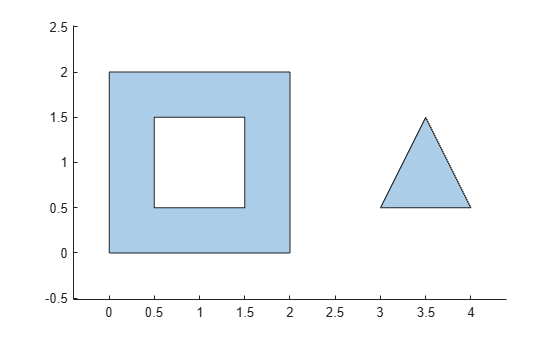# area

Area of `polyshape`

## Syntax

``A = area(polyin)``
``A = area(polyin,I)``

## Description

example

````A = area(polyin)` returns the total area of a `polyshape` object, which is the sum of the areas of the solid regions that make up the `polyshape`.```
````A = area(polyin,I)` returns the area of the `I`th boundary of `polyin`. The area of a hole boundary is negative.This syntax is only supported when `polyin` is a scalar `polyshape` object.```

## Examples

collapse all

Create a polygon containing multiple solid regions and one hole, and compute its area.

```P = [0 0; 0 2; 2 2; 2 0; NaN NaN; 0.5 0.5; 0.5 1.5; 1.5 1.5; 1.5 0.5; NaN NaN; 3 0.5; 3.5 1.5; 4 0.5]; polyin = polyshape(P); plot(polyin) axis equal````A = area(polyin)`
```A = 3.5000 ```

## Input Arguments

collapse all

Input `polyshape`, specified as a scalar, vector, matrix, or multidimensional array.

Data Types: `polyshape`

Boundary index, specified as a scalar integer or vector of integers. Each element of `I` corresponds to a single boundary of the input `polyshape`.

Data Types: `double` | `single` | `int8` | `int16` | `int32` | `int64` | `uint8` | `uint16` | `uint32` | `uint64`